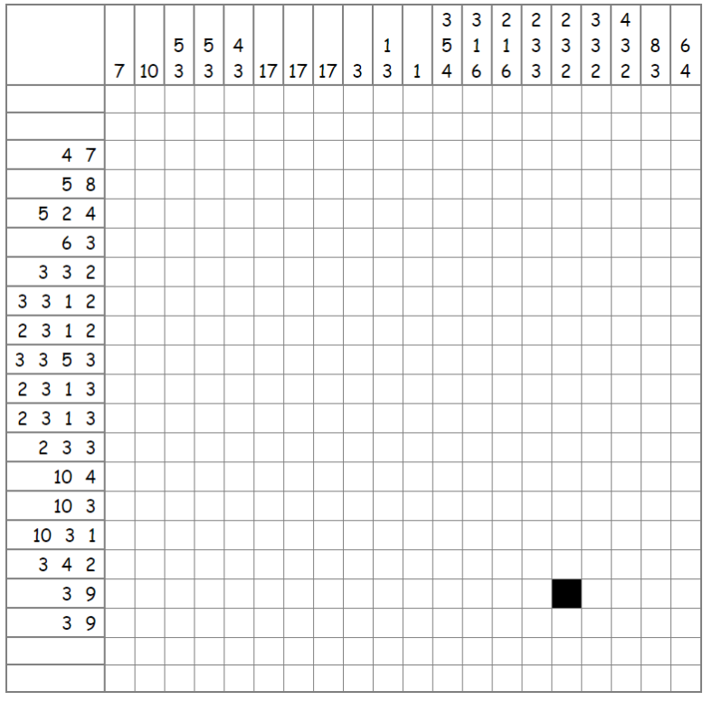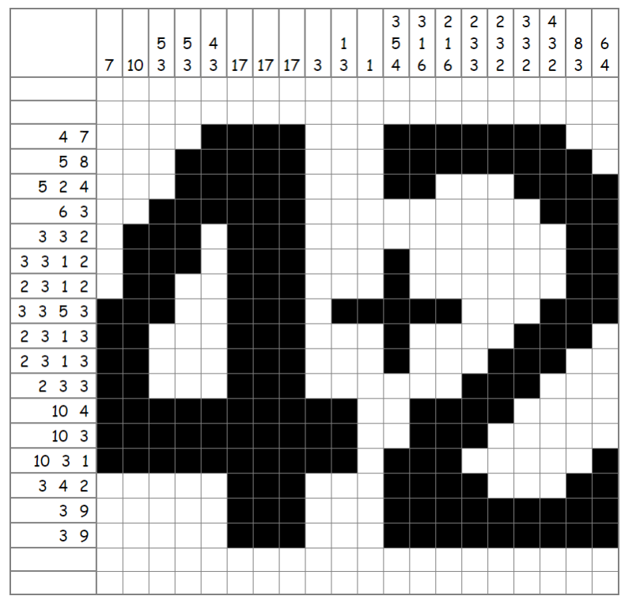Maths Challenge 7# Maths Challenge 7

## 1: Simple Sum...Logic

What is the answer to the below sum?Pdf version for printing
Excel version of puzzle grid

This is a Nonogram (also known as a Hanjie, Picross, Griddler or Pic-a-pix). For information on how to solve this type of logic puzzle you can visit this Wikipedia entry on Nonograms

There are 21 rows to the grid and 4 of them have on blocks coloured in leaving a height of 17 possible squares in any column. This means you can instantly fill in all the columns labelled as containing 17 black squares.Excel verion of the completed nonogram
The answer is 6

## 2: Angular RatioRatios and Basic TrigonometryThe angles of the above triangle are in the ratio 5:4:3 (a:b:c)

What is the size of each angle?

Remember the basic angle rule of triangles: All internal angles add up to a total of 180°

180 ÷ (5 + 4 + 3) = 180 ÷ 12 = 15
So, a = 5 × 15 = 75°
b = 4 × 15 = 60°
and c = 3 × 15 = 45°

## 3: Science LessonNumeracy

The Lab Technician needs to prepare enough materials for a class experiment.
Each pupil will need 95cm3 of vinegar
There are 20 pupils in the class
Vinegar comes in 1 litre bottles
How many whole bottles of vinegar will be needed?

Adapted from the Department for Education Numeracy Professional Skills Test: Practice Test 2

The answer will be a whole number

2, because 95 x 20 = 1900cm3 and the bottles are 1 litre bottles so 1 bottle will not be enough but 2 will cater for the whole class

## 4: Training PlanA-Level: Exponential Growth

An athlete plans a training schedule which involves running 20km in the first week of training; in each subsequent week the distance is to be increased by 10% over the previous week.
Write down an expression for the distance to be covered in the nth week according to this schedule, and find in which week the athlete would first cover more than 100km.

Use the basic exponential growth model of:

P = P0 [1 + x/100]t

Distance = 20(1.1)n-1
20(1.1)n-1 > 100
(1.1)n-1 >5
Using trial and error
(1.1)16 = 4.6
(1.1)17 = 5.05
so week 18 is the first time the athlete will exceed 100km.

## 5: Deciphering the AnswerBasic Operations

odmin nsoid veaiw neioh dxtuv dwuet sslet nriet edydm wb (7)

This links into the Transposition Ciphers Workshop

This is encrypted using the Rail Fence Cipher over 7 lines

The message reads:

one hundred and six times two minus twelve divided by two

So, what is the answer?
Note: Remember the order of Operations (Division, Multiplication, Addition, Subtraction)
106 × 2 - 12 ÷ 2
= 106 × 2 - 6
= 212 - 6
= 206